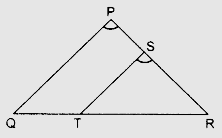# $S$ and $T$ are points on sides $PR$ and $QR$ of $ГўЛ†вЂ PQR$ such that $\angle P = \angle RTS$. Show that $ГўЛ†вЂ RPQ \sim ГўЛ†вЂ RTS$.

Given:

$S$ and $T$ are points on sides $PR$ and $QR$ of $∆PQR$ such that $\angle P = \angle RTS$.

To do:

We have to show that $∆RPQ \sim ∆RTS$.

Solution:In $\triangle RPQ$ and $\triangle RTS$,

$\angle P=\angle RTS$

$\angle P=\angle R$

Therefore, by AA criterion,

$\Delta RPQ \sim \Delta RTS$

Hence proved.

Updated on: 10-Oct-2022

38 Views